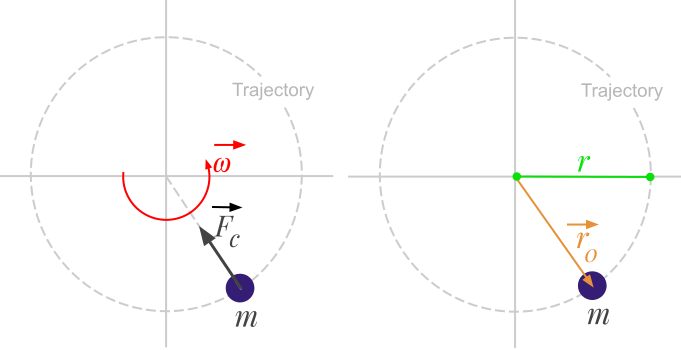👋 Hello! I'm Alphonsio the robot. Ask me a question, I'll try to answer.

# How to calculate the centripetal force based on angular velocity ?The centripetal force can be calculated with the following formula:

﻿﻿

The previous equation can be generalized in vector form:

﻿﻿

Where:

• ﻿﻿ is the centripetal force expressed in Newton (﻿﻿)
• ﻿﻿ is the body mass expressed in kilograms (﻿﻿)
• ﻿﻿ is the angular velocity of the body expressed in radians per second (﻿﻿)
• ﻿﻿ is the angular velocity vector
• ﻿﻿ is the radius of the circular path expressed in meters (﻿﻿)
• ﻿﻿ is a radius vector attached to the center of the circular path, in the direction of the body.
More